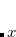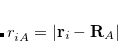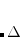Q-Chem 5.1 User’s Manual

# 11.10 Harmonic Vibrational Analysis

Vibrational analysis is an extremely important tool for the quantum chemist, supplying a molecular fingerprint which is invaluable for aiding identification of molecular species in many experimental studies. Q-Chem includes a vibrational analysis package that can calculate vibrational frequencies and their Raman and infrared activities. Vibrational frequencies are calculated by either using an analytic Hessian (if available; see Table 10.1) or, numerical finite difference of the gradient. The default setting in Q-Chem is to use the highest analytical derivative order available for the requested theoretical method.

When calculating analytic frequencies at the HF and DFT levels of theory, the coupled-perturbed SCF equations must be solved. This is the most time-consuming step in the calculation, and also consumes the most memory. The amount of memory required iswhereis the number of basis functions, andthe number of atoms. This is an order more memory than is required for the SCF calculation, and is often the limiting consideration when treating larger systems analytically. Q-Chem incorporates a new approach to this problem that avoids this memory bottleneck by solving the CPSCF equations in segments. Instead of solving for all the perturbations at once, they are divided into several segments, and the CPSCF is applied for one segment at a time, resulting in a memory scaling of, whereis the number of segments. This option is invoked automatically by the program.

Following a vibrational analysis, Q-Chem computes useful statistical thermodynamic properties at standard temperature and pressure, including: zero-point vibration energy (ZPVE) and, translational, rotational and vibrational, entropies and enthalpies.

The performance of various ab initio theories in determining vibrational frequencies has been well documented; see Refs. Murray:1992,Scott:1996,Johnson:1993d.

## 11.10.1 Job Control

In order to carry out a frequency analysis users must at a minimum provide a molecule within the $molecule keyword and define an appropriate level of theory within the$rem keyword using the $rem variables EXCHANGE, CORRELATION (if required) (Chapter 4) and BASIS (Chapter 8). Since the default type of job (JOBTYPE) is a single point energy (SP) calculation, the JOBTYPE$rem variable must be set to FREQ.

It is very important to note that a vibrational frequency analysis must be performed at a stationary point on the potential surface that has been optimized at the same level of theory. Therefore a vibrational frequency analysis most naturally follows a geometry optimization in the same input deck, where the molecular geometry is obtained (see examples).

Users should also be aware that the quality of the quadrature grid used in DFT calculations is more important when calculating second derivatives. The default grid for some atoms has changed in Q-Chem 3.0 (see Section 5.5) and for this reason vibrational frequencies may vary slightly form previous versions. It is recommended that a grid larger than the default grid is used when performing frequency calculations.

The standard output from a frequency analysis includes the following.

• Vibrational frequencies.

• Raman and IR activities and intensities (requires $rem DORAMAN). • Atomic masses. • Zero-point vibrational energy. • Translational, rotational, and vibrational, entropies and enthalpies. Several other$rem variables are available that control the vibrational frequency analysis. In detail, they are:

DORAMAN
 Controls calculation of Raman intensities. Requires JOBTYPE to be set to FREQ

TYPE:
 LOGICAL

DEFAULT:
 FALSE

OPTIONS:
 FALSE Do not calculate Raman intensities. TRUE Do calculate Raman intensities.

RECOMMENDATION:
 None

VIBMAN_PRINT
 Controls level of extra print out for vibrational analysis.

TYPE:
 INTEGER

DEFAULT:
 1

OPTIONS:
 1 Standard full information print out. If VCI is TRUE, overtones and combination bands are also printed. 3 Level 1 plus vibrational frequencies in atomic units. 4 Level 3 plus mass-weighted Hessian matrix, projected mass-weighted Hessian matrix. 6 Level 4 plus vectors for translations and rotations projection matrix.

RECOMMENDATION:
 Use the default.

CPSCF_NSEG
 Controls the number of segments used to calculate the CPSCF equations.

TYPE:
 INTEGER

DEFAULT:
 0

OPTIONS:
 0 Do not solve the CPSCF equations in segments.User-defined. Usesegments when solving the CPSCF equations.

RECOMMENDATION:
 Use the default.

Example 11.254  An EDF1/6-31+G* optimization, followed by a vibrational analysis. Doing the vibrational analysis at a stationary point is necessary for the results to be valid.

$molecule 0 1 O C 1 co F 2 fc 1 fco H 2 hc 1 hco 3 180.0 co = 1.2 fc = 1.4 hc = 1.0 fco = 120.0 hco = 120.0$end

$rem JOBTYPE opt METHOD edf1 BASIS 6-31+G*$end

@@@

$molecule read$end

$rem JOBTYPE freq METHOD edf1 BASIS 6-31+G*$end


## 11.10.2 Isotopic Substitutions

By default Q-Chem calculates vibrational frequencies using the atomic masses of the most abundant isotopes (taken from the Handbook of Chemistry and Physics,Edition). Masses of other isotopes can be specified using the $isotopes section and by setting the ISOTOPES$rem variable to TRUE. The format of the $isotopes section is as follows: $isotopes
number_of_isotope_loops  tp_flag
number_of_atoms  [temp pressure] (loop 1)
atom_number1   mass1
atom_number2   mass2
...
number_of_atoms  [temp pressure] (loop 2)
atom_number1   mass1
atom_number2   mass2
...
$end  Note: Only the atoms whose masses are to be changed from the default values need to be specified. After each loop all masses are reset to the default values. Atoms are numbered according to the order in the$molecule section.

An initial loop using the default masses is always performed first. Subsequent loops use the user-specified atomic masses. Only those atoms whose masses are to be changed need to be included in the list, all other atoms will adopt the default masses. The output gives a full frequency analysis for each loop. Note that the calculation of vibrational frequencies in the additional loops only involves a rescaling of the computed Hessian, and therefore takes little additional computational time.

The first line of the $isotopes section specifies the number of substitution loops and also whether the temperature and pressure should be modified. The tp_flag setting should be set to 0 if the default temperature and pressure are to be used (298.18 K and 1 atm respectively), or else to 1 if they are to be altered. Note that the temperatures should be specified in Kelvin and pressures in atmospheres. ISOTOPES  Specifies if non-default masses are to be used in the frequency calculation. TYPE:  LOGICAL DEFAULT:  FALSE OPTIONS:  FALSE Use default masses only. TRUE Read isotope masses from$isotopes section.

RECOMMENDATION:
 None

Example 11.255  An EDF1/6-31+G* optimization, followed by a vibrational analysis. Doing the vibrational analysis at a stationary point is necessary for the results to be valid.

$molecule 0 1 C 1.08900 0.00000 0.00000 C -1.08900 0.00000 0.00000 H 2.08900 0.00000 0.00000 H -2.08900 0.00000 0.00000$end

$rem BASIS 3-21G JOBTYPE opt METHOD hf$end

@@@

$molecule read$end

$rem BASIS 3-21G JOBTYPE freq METHOD hf SCF_GUESS read ISOTOPES 1$end

$isotopes 2 0 ! two loops, both at std temp and pressure 4 1 13.00336 ! All atoms are given non-default masses 2 13.00336 3 2.01410 4 2.01410 2 3 2.01410 ! H's replaced with D's 4 2.01410$end


## 11.10.3 Partial Hessian Vibrational Analysis

The computation of harmonic frequencies for systems with a very large number of atoms can become computationally expensive. However, in many cases only a few specific vibrational modes or vibrational modes localized in a region of the system are of interest. A typical example is the calculation of the vibrational modes of a molecule adsorbed on a surface. In such a case, only the vibrational modes of the adsorbate are useful, and the vibrational modes associated with the surface atoms are of less interest. If the vibrational modes of interest are only weakly coupled to the vibrational modes associated with the rest of the system, it can be appropriate to adopt a partial Hessian approach. In this approach, only the part of the Hessian matrix comprising the second derivatives of a subset of the atoms defined by the user is computed. These atoms are defined in the $alist block. This results in a significant decrease in the cost of the calculation. Physically, this approximation corresponds to assigning an infinite mass to all the atoms excluded from the Hessian and will only yield sensible results if these atoms are not involved in the vibrational modes of interest. VPT2 and TOSH anharmonic frequencies can be computed following a partial Hessian calculation. It is also possible to include a subset of the harmonic vibrational modes with an anharmonic frequency calculation by invoking the ANHAR_SEL rem. This can be useful to reduce the computational cost of an anharmonic frequency calculation or to explore the coupling between specific vibrational modes. Alternatively, vibrationally averaged interactions with the rest of the system can be folded into a partial Hessian calculation using vibrational subsystem analysis. Based on an adiabatic approximation, this procedure reduces the cost of diagonalizing the full Hessian, while providing a local probe of fragments vibrations, and providing better than partial Hessian accuracy for the low frequency modes of large molecules. Mass-effects from the rest of the system can be vibrationally averaged or excluded within this scheme. PHESS  Controls whether partial Hessian calculations are performed. TYPE:  INTEGER DEFAULT:  0 Full Hessian calculation OPTIONS:  1 Partial Hessian calculation. 2 Vibrational subsystem analysis (massless). 3 Vibrational subsystem analysis (weighted). RECOMMENDATION:  None N_SOL  Specifies number of atoms included in the Hessian. TYPE:  INTEGER DEFAULT:  No default OPTIONS:  User defined RECOMMENDATION:  None PH_FAST  Lowers integral cutoff in partial Hessian calculation is performed. TYPE:  LOGICAL DEFAULT:  FALSE Use default cutoffs OPTIONS:  TRUE Lower integral cutoffs RECOMMENDATION:  None ANHAR_SEL  Select a subset of normal modes for subsequent anharmonic frequency analysis. TYPE:  LOGICAL DEFAULT:  FALSE Use all normal modes OPTIONS:  TRUE Select subset of normal modes RECOMMENDATION:  None Example 11.256 This example shows an anharmonic frequency calculation for ethene where only the C-H stretching modes are included in the anharmonic analysis. $comment
ethene
restricted anharmonic frequency analysis
$end$molecule
0 1
C   0.6665   0.0000   0.0000
C  -0.6665   0.0000   0.0000
H   1.2480   0.9304   0.0000
H  -1.2480  -0.9304   0.0000
H  -1.2480   0.9304   0.0000
H   1.2480  -0.9304   0.0000
$end$rem
JOBTYPE           freq
METHOD            hf
BASIS             sto-3g
ANHAR_SEL         TRUE
N_SOL             4
$end$alist
9
10
11
12
$end  Example 11.257 This example shows a partial Hessian frequency calculation of the vibrational frequencies of acetylene on a model of the C(100) surface $comment
acetylene - C(100)
partial Hessian calculation
$end$molecule
0 1
C  0.000  0.659 -2.173
C  0.000 -0.659 -2.173
H  0.000  1.406 -2.956
H  0.000 -1.406 -2.956
C  0.000  0.786 -0.647
C  0.000 -0.786 -0.647
C  1.253  1.192  0.164
C -1.253  1.192  0.164
C  1.253 -1.192  0.164
C  1.297  0.000  1.155
C -1.253 -1.192  0.164
C  0.000  0.000  2.023
C -1.297  0.000  1.155
H -2.179  0.000  1.795
H -1.148 -2.156  0.654
H  0.000 -0.876  2.669
H  2.179  0.000  1.795
H -1.148  2.156  0.654
H -2.153 -1.211 -0.446
H  2.153 -1.211 -0.446
H  1.148 -2.156  0.654
H  1.148  2.156  0.654
H  2.153  1.211 -0.446
H -2.153  1.211 -0.446
H  0.000  0.876  2.669
$end$rem
JOBTYPE           freq
METHOD            hf
BASIS             sto-3g
PHESS             TRUE
N_SOL             4
$end$alist
1
2
3
4
$end  ## 11.10.4 Localized Mode Vibrational Analysis The computation of harmonic frequencies leads to molecular vibrations described by coordinates which are often highly de-localized. For larger molecules many vibrational modes can potentially contribute to a single observed spectral band, and information about the interaction between localized chemical units can become less readily available. In certain cases, localizing vibrational modes using procedures similar to the localized orbital schemes discussed previously in this manual can therefore provide a more chemically intuitive way of analysing spectral data, interpreting two-dimensional vibrational spectra, or improving calculations that go beyond the harmonic approximation. It is also possible to include only a subset of the normal modes in the localization calculation by invoking the LOCALFREQ_SELECT rem variable. This can be useful to improve convergence in larger molecules or to explore the coupling between specific vibrational modes. These modes are defined in the$alist block. Alternatively it is possible to localize high and low frequency modes separately in a single calculation using LOCALFREQ_GROUPS and related inputs.

LOCAL_FREQ
 Controls whether a vibrational mode localization calculation is performed.

TYPE:
 INTEGER

DEFAULT:
 0 Normal mode calculation.

OPTIONS:
 1 Localized mode calculation with a Pipek-Mezey like criterion. 2 Localized mode calculation with a Boys like criterion.

RECOMMENDATION:
 None

LOCALFREQ_THRESH
 Mode localization is considered converged when the change in the localization criterion is less than.

TYPE:
 INTEGER

DEFAULT:
 6

OPTIONS:User-specified integer.

RECOMMENDATION:
 None

LOCALFREQ_MAX_ITER
 Controls the maximum number of mode localization sweeps permitted.

TYPE:
 INTEGER

DEFAULT:
 200

OPTIONS:User-specified integer.

RECOMMENDATION:
 None

LOCALFREQ_SELECT
 Select a subset of normal modes for subsequent anharmonic frequency analysis.

TYPE:
 LOGICAL

DEFAULT:
 FALSE Use all normal modes.

OPTIONS:
 TRUE Select a subset of normal modes.

RECOMMENDATION:
 None

LOCALFREQ_GROUPS
 Select the number of groups of frequencies to be localized separately within a localized mode calculation. The size of the groups are then controlled using the LOCALFREQ_GROUP1, LOCALFREQ_GROUP2, and LOCALFREQ_GROUP3 keywords.

TYPE:
 INTEGER

DEFAULT:
 0 Localize all normal modes together.

OPTIONS:
 1 Define one subset of modes to localize independently. 2 Define two subsets of modes to localize independently. 3 Define three subsets of modes to localize independently.

RECOMMENDATION:
 None

LOCALFREQ_GROUP1
 Select the number of modes to include in the first subset of modes to localize independently when the keyword LOCALFREQ_GROUPS > 0.

TYPE:
 INTEGER

DEFAULT:
 NONE

OPTIONS:User-specified integer.

RECOMMENDATION:
 Modes will be included starting with the lowest frequency mode and then in ascending energy order up to the defined value.

LOCALFREQ_GROUP2 and LOCALFREQ_GROUP3 are defined similarly.